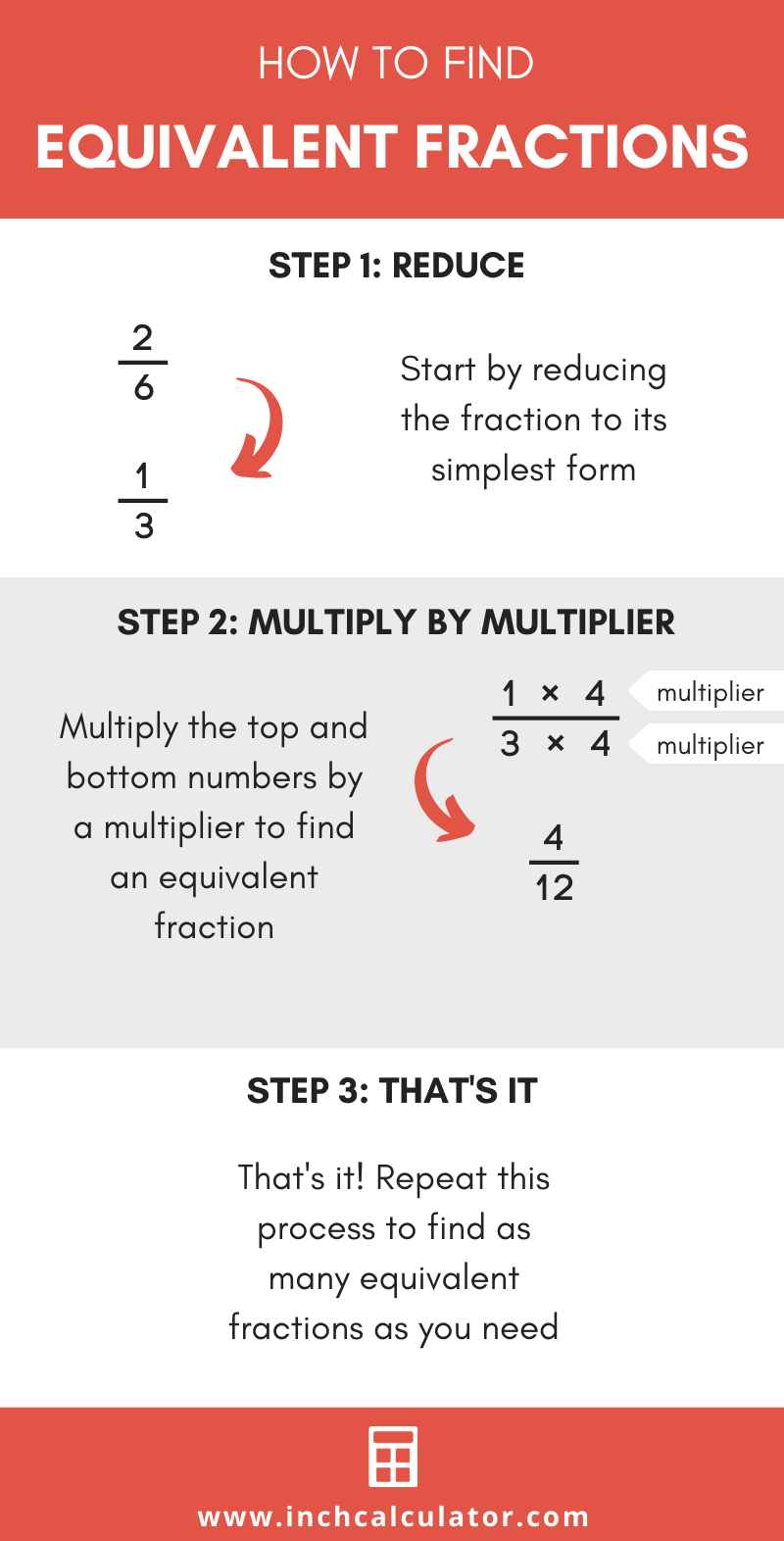# Equivalent Fractions Calculator

Calculate equivalent fractions for a proper, improper, or mixed fraction or whole number using the calculator below. The results include the first 100 equivalent fractions.

## Equivalent Fractions:

 4/6 6/9 8/12 10/15 12/18 14/21 16/24 18/27 20/30 22/33 24/36 26/39 28/42 30/45 32/48 34/51 36/54 38/57 40/60 42/63 44/66 46/69 48/72 50/75 52/78 54/81 56/84 58/87 60/90 62/93 64/96 66/99 68/102 70/105 72/108 74/111 76/114 78/117 80/120 82/123 84/126 86/129 88/132 90/135 92/138 94/141 96/144 98/147 100/150 102/153 104/156 106/159 108/162 110/165 112/168 114/171 116/174 118/177 120/180 122/183 124/186 126/189 128/192 130/195 132/198 134/201 136/204 138/207 140/210 142/213 144/216 146/219 148/222 150/225 152/228 154/231 156/234 158/237 160/240 162/243 164/246 166/249 168/252 170/255 172/258 174/261 176/264 178/267 180/270 182/273 184/276 186/279 188/282 190/285 192/288 194/291 196/294 198/297 200/300 202/303
Learn how we calculated this below

## How to Calculate Equivalent Fractions

Equivalent fractions are fractions equal in value to another but that have a different numerator and denominator. There are a few steps to find equivalent fractions.

### Step One: Reduce

The first step to find equivalent fractions is to simplify the starting fraction to its reduced form.

Find the greatest common factor of the top and bottom numbers, then divide both by the greatest common factor to reduce.

For example, let’s find an equivalent fraction for 6/9 by reducing.

The greatest common factor of 6 and 9 is 3 (3 is the largest number that evenly goes into 6 and 9).

6 ÷ 3 = 2
9 ÷ 3 = 3

So, 2/3 is equivalent to 6/9.

### Step Two: Calculate Equivalent Fractions

The second step is to calculate fractions that are equal in value to the original. Multiply the numerator and denominator of the reduced fraction by a multiplier to find an equivalent fraction.

For example, let’s find some equivalent fractions for 2/6 by reducing and then multiplying.

Reduce the fraction first.

2 / 6 = 1 / 3

Multiply the top and bottom numbers of 1/3 by the multiplier 4.

1 × 4 / 3 × 4
4 / 12

Thus, 4/12 is an equivalent fraction of 1/3.Another easy way to find equivalent fractions is to use an equal fractions chart.

## How to Find if Two Fractions are Equivalent

There are three ways to find if two fractions are equivalent to each other.

The first method is to reduce each fraction to the simplest form. If the reduced fractions are equal, then the fractions are equivalent.

For example, let’s find out if 5/10 and 6/12 are equivalent.

We can simplify 5/10 by dividing by the greatest common factor, 5.

5 ÷ 5 = 1
10 ÷ 5 = 2

1/2 is equivalent to 5/10 in simplest form.

We can simplify 6/12 by dividing by the greatest common factor, 6.

6 ÷ 6 = 1
12 ÷ 6 = 2

1/2 is 6/12 in simplest form.

5/10 and 6/12 simplified are both equal to 1/2. Therefore, the fractions are equivalent.

The second method is to convert each fraction to a decimal by dividing the top number of each by the bottom. If the decimal values are equal, then the fractions are equivalent.

For example, we can find out if 5/10 and 6/12 are equivalent by dividing the numerator by the denominator.

5 ÷ 10 = 0.5
6 ÷ 12 = 0.5

Since the decimals are the same, we know that the fractions are equivalent.

The third method is to use a fraction comparing calculator to determine if the fractions are equivalent automatically.